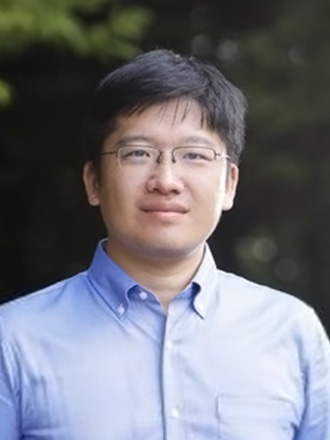职称： 教授，博导，国家级高层次人才计划入选者 基础数学系 闵行数学楼322室 54342646-322 bliu@math.ecnu.edu.cn http://math.ecnu.edu.cn/~bliu https://faculty.ecnu.edu.cn/_s42/lb2/main.psp

Resume and papers

Research field

Differential Geometry.

Special interests

Global analysis on manifolds, local index theory and differential K-theory.

Main research objects

Analytic and differential-topological properties of Atiyah-Patodi-Singer eta-invariant, Bismut-Cheeger eta form, Ray-Singer analytic torsion, elliptic genera and related objects, especially on relations between eta forms and differential K-theory.

Employment history
Postdoc: Universität zu Köln; Humboldt-Universität zu Berlin in Germany.

Visit
2017.1 Institut des Hautes Études Scientifiques (IHES), France;
2017.3 Max Planck Institute for Mathematics (MPIM), Germany;
2017.5 University of California, Santa Barbara, USA
2018.5 Institut de Mathematiques de Jussieu, France.

Education

2013.12 Ph.D., Mathematics, Chern Institute of Mathematics, Nankai University of China. (Advisor: Prof. Weiping Zhang)

Publications
 (with Jianqing Yu) On the Anomaly Formula for the Cappell-Miller Holomorphic Torsion. Sci. China Math.. 2010, 53(12): 3225-3241.
 (with Jianqing Yu) On the Witten Rigidity Theorem for Stringc Manifolds. Pacific J. Math., 2013, 266(2): 477-508.
 (with Jianqing Yu) Rigidity and Vanishing Theorems on Z/k Spinc manifolds. Trans. Amer. Math. Soc. 2015, 367(2), 1381–1420.
 Functoriality of Equivariant Eta Forms. Journal of Noncommutative Geometry. 2017, 11(1), 225-307.
 Real embedding and Equivariant Eta Forms. Math. Z. 292 (2019), 849-878.
 (with Xiaonan Ma) Differential K-theory, eta-invariant, and localization. C. R. Math. Acad. Sci. Paris. 357(10) (2019), 803--813.
(with Xiaonan Ma) Differential K-theory and localization formula of eta invariants. Invent. Math. 222(2) (2020), 545-613.
 Equivariant eta forms and equivariant differential K-theory. Sci. China Math. 64(10) (2021), 2159-2206.
 (with Xiaonan Ma) Comparison of two equivariant eta forms. 61 pages. arXiv:1808.04044. To appear in Advances in Mathematics

Preprints
 Bismut-Cheeger eta forms and higher spectral flow. arXiv:2105.01926.

Notes

 Complex manifold and Kaehler geometry (2018 spring course)

 Global analysis on manifolds (2019 spring course)

Teaching:

Autumn 2019: Mathematical Analysis I

Spring 2020: Mathematical Analysis II

Autumn 2020: Mathematical Analysis III

Spring 2021: Differential Geometry

Spring 2022: Differential Geometry